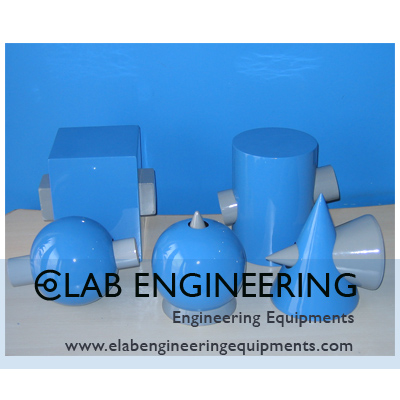# A Set of Wooden Model on Interpenetration of Solids [Code: ODM06]Description & Specification:

A Set of Wooden Model on Interpenetration of Solids.

(A) Two cylinders of different diameters penetrating each other at right angles.

(B) Two cylinders of different diameters with oblique penetration.

(C) A cylinder into a cone with oblique penetration.

(D) A Cone and cylinder with penetration at right angles.

(E) Two cones at right angles.

(F) Two square prisms of different sizes at right angles.

(G) Two square prisms at oblique angles.

(H) Sphere and cone at centre.

(I) Sphere and cylinder eccentric.

(J) Sphere and cylinder at centre.

Object Drawing Models,A Set of Wooden Model on Interpenetration of Solids